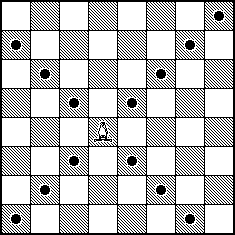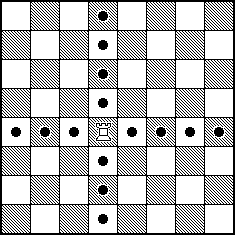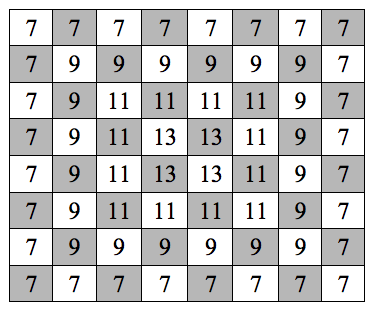## Exercise 1

Just before their last year at Anytown High School, the seniors hold the Senior Year Kick-Off party. Sixty percent of the students attending the party are seniors, and the rest (friends, significant others, siblings, etc.) are juniors (25%), sophomores (10%), and freshmen (5%) Unfortunately, drinking is quite common at this party; 90% of the seniors consume alcohol, so do 80% of the juniors, 50% of the sophomores, and 20% of the freshmen.

(a) If a student at this party is drinking, what is the probability that this student is a senior?

Solution:

First, we note the given information

\begin{aligned} P(Fr) &= 0.05, \ P(D \mid Fr) = 0.20 \\ P(So) &= 0.10, \ P(D \mid So) = 0.50 \\ P(Jr) &= 0.25, \ P(D \mid Jr) = 0.80 \\ P(Sr) &= 0.60, \ P(D \mid Sr) = 0.90 \\ \end{aligned}

Next, we calculate proportion of the students attending the party consume alcohol.

\begin{aligned} P(D) &= P(Fr \cap D) + P(So \cap D) + P(Jr \cap D) + P(Sr \cap D) \\ &= P(Fr) \cdot P(D \mid Fr) + P(So) \cdot P(D \mid So) + P(Jr) \cdot P(D \mid Jr) + P(Sr) \cdot P(D \mid Sr) \\ &= 0.05 \cdot 0.20 + 0.10 \cdot 0.50 + 0.25 \cdot 0.80 + 0.60 \cdot 0.90 \\ &= 0.01 + 0.05 + 0.20 + 0.54 \\ &= 0.80 \end{aligned}

Note that, along the way we obtained the probability of drinking and the intersection with each class.

Then, we finally calculate

$P(Sr \mid D) = \frac{P(Sr \cap D) }{P(D)} = \frac{0.54}{0.80} = \boxed{0.675}$

(b) If a student at this party is not drinking, what is the probability that this student is not a senior?

Solution:

Based on the work we did in the previous problem, we can easily “complete the table.” (We could have also used a tree to get the information needed for the table.)

Fr So Jr Sr
Drinking 0.01 0.05 0.20 0.54 0.80
Not Drinking 0.04 0.05 0.05 0.06 0.20
0.05 0.10 0.25 0.60 1

$P(Sr^\prime \mid D^\prime) = \frac{P(Sr^\prime \cap D^\prime)}{P(D^\prime)} = \frac{0.04 + 0.05 + 0.05}{0.20} = \boxed{0.70}$

(c) If a student at this party is not a senior, what is the probability that this student is not drinking?

Solution:

$P(D^\prime \mid Sr^\prime) = \frac{P(D^\prime \cap Sr^\prime)}{P(Sr^\prime)} = \frac{0.04 + 0.05 + 0.05}{0.05 + 0.10 + 0.25} = \boxed{0.35}$

(d) What proportion of the underclassmen (freshmen and sophomores) attending the party consume alcohol?

Solution:

$P(D \mid (Fr \cup So)) = \frac{0.01 + 0.05}{0.05 + 0.10} = \boxed{0.40}$

(e) The school administration discourages the Senior Year Kick-Off party; the principal of AHS announced that any senior attending the party will receive a week of detention. Of course, drinking is also discouraged. Find the proportion of the students at the party who either are seniors, or consume alcohol, or both.

Solution:

$P(S \cup D) = P(S) + P(D) - P(S \cap D) = 0.60 + 0.80 - 0.54 = \boxed{0.86}$

(f) Are events {a student at the party is a senior} and {a student at the party is drinking} independent? Justify your answer. No credit will be given without proper justification.

Solution:

$$\boxed{\text{No.}}$$

$0.54 = P(Sr \cap D) \neq P(Sr) \cdot P(D) = 0.60 \cdot 0.80 = 0.48$

We could have also showed that $$P(Sr \mid D) \neq P(Sr)$$ or $$P(D \mid Sr) \neq P(D)$$.

(g) Are events {a student at the party is a junior} and {a student at the party is drinking} independent? Justify your answer. No credit will be given without proper justification.

Solution:

$$\boxed{\text{Yes.}}$$

$0.20 = P(Jr \cap D) \neq P(Jr) \cdot P(D) = 0.25 \cdot 0.80 = 0.20$

## Exercise 2

A bishop is placed at random (with equal chance) on a chess board (8 x 8). A king of the opposing color is placed at random (with equal chance) on one of the remaining squares. What is the probability that the king is under attack from the bishop?Bishop: possible attacks.

Hint: Placed anywhere on a chess board, a rook attacks 14 squares out of the remaining 63.Rook: possible attacks.

$P(\text{ K is under attack }) = \frac{14}{63}$

Solution:

We first need to determine how many squares are being attacked for each possible position of the bishop. For example, in the top left corner, the bishop can attack 7 positions. We also keep track of how many positions on the board have the bishop attacking 7 positions. (And do so for all possibilities.)Bishop: number of positions attacked from each position.

Then we use the law of total probability.

\begin{aligned} P(\text{K is under attack}) &= P(\text{B attacks 7}) \cdot P(\text{K is under attack} \mid \text{B attacks 7}) \\ &+ P(\text{B attacks 9}) \cdot P(\text{K is under attack} \mid \text{B attacks 9}) \\ &+ P(\text{B attacks 11}) \cdot P(\text{K is under attack} \mid \text{B attacks 11}) \\ &+ P(\text{B attacks 13}) \cdot P(\text{K is under attack} \mid \text{B attacks 13}) \\ &= \frac{28}{64} \cdot \frac{7}{63} + \frac{20}{64} \cdot \frac{9}{63} + \frac{12}{64} \cdot \frac{11}{63} + \frac{4}{64} \cdot \frac{13}{63} \\ &= \boxed{\frac{5}{36}} \end{aligned}

# Exercise 3

You are given that $$P(A) = 0.5$$ and $$P(A \cup B) = 0.7$$. Student 1 assumes that $$A$$ and $$B$$ are independent and calculates $$P(B)$$ based on that assumption. Student 2 assumes that $$A$$ and $$B$$ are mutually exclusive and calculates $$P(B)$$ based on that assumption. Find the absolute difference between the two calculations.

Solution:

We first consider Student 1. Since Student 1 believes that $$A$$ and $$B$$ are independent, we have

$P_1(A \cap B) = P_1(A) \cdot P_1(B)$

It is also always true that

$P_1(A \cup B) = P_1(A) + P_1(B) - P_1(A \cap B)$

With the given probabilities, we have

\begin{aligned} P_1(A \cap B) &= 0.5 \cdot P_1(B) \\ 0.7 &= 0.5 + P_1(B) - P_1(A \cap B) \end{aligned}

This is a system of two equation, with two unknowns, so we solve and obtain

$P_1(B) = 0.40$

Now we consider Student 2. Since they assumes that $$A$$ and $$B$$ are mutually exclusive we have

$P_2(A \cap B) = 0.$

Because of this, we have

$P_2(A \cup B) = P_2(A) + P_2(B)$

With the given probabilities, we have

$0.7 = 0.5 + P_2(B)$

So we obtain

$P_2(B) = 0.2.$

So, finally, their absolute difference is

$\left| P_1(B) - P_2(B)\right | = 0.4 - 0.2 = \boxed{0.2}$

# Exercise 4

Alex and David agreed to play a series of tennis games (as many as needed) until one of them wins two games in a row. Alex will serve in the first game, then the serve would alternate game by game between Alex and David. David is a better tennis player; Alex has a 50% chance of winning a game on his serve and only a 20% chance of winning a game if David serves. Assume that all games are independent. Find the probability that Alex is the first one to win two games in a row.

Solution:

First we define some notation. We say

$O_S$

$$O$$ is the outcome for Alex, $$W$$ or $$L$$. $$S$$ is the player serving. $$D$$ for David, $$A$$ for Alex.

Now we enumerate the possible outcomes that result in Alex being the first to win two games in a row. Recall that Alex serves first. We can group these into two groups of possibilities.

Alex wins the first game:

\begin{aligned} & W_{A}W_{D} \\ & W_{A}L_{D} W_{A}W_{D} \\ & W_{A}L_{D} W_{A}L_{D} W_{A}W_{D} \\ & \ldots \end{aligned}

David wins the first game:

\begin{aligned} & L_{A}W_{D}W_{A} \\ & L_{A}W_{D} L_{A}W_{D}W_{A} \\ & L_{A}W_{D} L_{A}W_{D} L_{A}W_{D}W_{A} \\ & \ldots \end{aligned}

Or, more succinctly:

Alex wins first game: $$(W_{A}L_{D})^k W_{A}W_{D}, \quad k = 0, 1, 2, 3, \ldots$$

David wins first game: $$L_{A} (W_{D}L_{A})^k W_{D}W_{A}, \quad k = 0, 1, 2, 3, \ldots$$

Then, we calculate the required probability by adding the probabilities of each of the outcomes we listed above, since they are all disjoint.

\begin{aligned} P(\text{ Alex wins two in a row }) &= P(\text{ Alex wins two in a row after winning the first }) \\[0.5em] &+ P(\text{ Alex wins two in a row after losing the first }) \\[0.5em] &= \sum_{k = 0}^{\infty}(0.50 \cdot 0.80)^k (0.50 \cdot 0.20) \\[0.5em] &+ \sum_{k = 0}^{\infty} 0.50 \cdot (0.20 \cdot 0.50)^k (0.20 \cdot 0.50) \\[0.5em] &= \frac{0.50 \cdot 0.20}{1 - 0.50 \cdot 0.80} = \frac{0.50 \cdot 0.20 \cdot 0.50}{1 - 0.20 \cdot 0.50} \\[0.5em] &= \frac{1}{6} + \frac{1}{18}\\[0.5em] &= \boxed{\frac{2}{9}} \end{aligned}